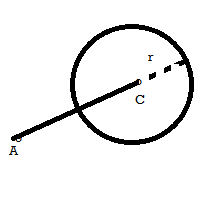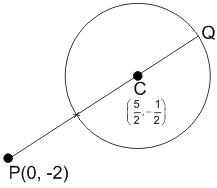# If a point P has co-ordinates (0, -2) and Q is any point on the circle, x2 + y2 - 5x - y + 5 = 0,then the maximum value of (PQ)2 is : Option 1)Option 2)Option 3)Option 4)N neha
V Vakul

As we learnt in

Greatest distance of a point A from a circle -

- whereinA is the point and circle has centre C and radius r.Q is point on the circle

PQ=Maximum distance =PC + r

Option 1)This option is incorrect

Option 2)This option is correct

Option 3)This option is incorrect

Option 4)This option is incorrect

Exams
Articles
Questions